Latest Banking jobs   »   Quantitative Aptitude Quiz For RRB PO...

# Quantitative Aptitude Quiz For RRB PO Mains 2021- 1st September

Directions (1-5): The following questions are accompanied by three statements (I), (II), and (III). You have to determine which statement(s) is/are sufficient /necessary to answer the questions

Q1. Find out the two-digits number, if the tens place digit is greater than unit place digit.
I. Sum of digits of the number is 9.
II. Ratio of number and interchanged number is 7 : 4
III. If the digits are interchanged then difference between interchanged number formed and original number is 27.
(a) cannot be answered even including all statement
(b) Only II and III
(c) All together are required
(d) Any two are required
(e) Either II or I or III.

Q2. Arun borrowed a sum which is compounded annually, what is the amount he returned in 2 years?
I. Rate of interest is 6% per annum
II. Simple interest incurred on the sum in one year is Rs. 600
III. The borrowed sum is 10 times the amount earned as simple interest in two years.
(a) Only statement I and III are sufficient
(b) Only statement II is sufficient
(c) Only statement III is sufficient
(d) Any two are sufficient
(e) Statement II and either I or III are sufficient

Q3. What is speed of boat in still water?
I. speed of stream is two-third of speed of boat in still water
II. The boat covers 20 km in 2 hours in downstream
III. The boat covers 10 km in 5 hours in upstream.
(a) only statement II is sufficient
(b) Any two are sufficient
(c) I and II or III are sufficient
(d) Only statement III is sufficient
(e) None of these

Q4. What was the amount of profit earned?
I. If no discount is given, profit would be 40%
II. 30% discount is offered on marked price.
III. Selling price is more than cost price by 40%.
(a) Only III
(b) All I, II and III
(c) Only II and III
(d) cannot be answered even including all statement
(e) Only I and III

Q5. What is speed of train?
I. The train crosses 300 m long platform in 45 seconds.
II. The train crosses another stationary train of same length in 60 seconds.
III. The train crosses a single pole in 30 seconds.
(a) I and II or III
(b) Only I
(c) II and III both
(d) Only III
(e) I and II both

Directions (6-10): The following questions are accompanied by three statements (A), (B), and (C). You have to determine which statement(s) is/are sufficient /necessary to answer the questions
Q6. What is the speed of stream?
A) distance covered by boat against the stream in 20h is 200 km
B) distance covered by boat in downstream in 20h is 400 km
C) speed of boat in downstream is 200% of the speed of boat in upstream
(a) Only A and B together
(b) Either A and C or B and C
(c) Any two of them
(d) Either B alone or A and C together
(e) All statements are required

Q7. A boat takes 2 hours to travel from point A to B in still water. To find out the speed upstream, which of the following information is/are required?
A. Distance between point A and B.
B. Time taken to travel downstream from B to A.
C. Speed of the stream of water.
(a) All are required
(b) Any one pair of A and B, B and C or C and A is sufficient.
(c) Only A and B
(d) Only A and C
(e) None of these

Q8. What will be total surface area of the given diagram consisting one hemisphere & one cone?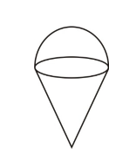A) Curved surface area of hemisphere is ‘x’ cm²
B) Volume of the given diagram is ‘y’ cm².
C) Ratio of height of cone to radius of hemisphere is 1 : 3
(a) Only A and B together
(b) Either A and B or B and C
(c) All statements are required
(d) Either B alone or A and C together
(e) Any two of them

Q9. In how much time A and B together can complete the whole work?
A) A and B can complete the work working alternatively starting from A in 16 days
B) Ratio of efficiency of A and B is 2 : 1
C) C can complete the work alone is 36 days while A and C together can complete the work in 9 days
(a) Only A and B together
(b) Either A and B or B and C
(c) Either A alone or B and C together
(d) Either B alone or A and C together
(e) Any two of them

Q10. Find out the two-digit given number?
A) If digits are interchanged then difference between new number formed and previous number is 36.
B) Sum of digits of the number is 12
C) Ratio of the number and interchanged number is 4 : 7
(a) Only A and B together
(b) Any two of them
(c) Either A and B or B and C
(d) All statements are required
(e) Either B alone or A and C together

Solutions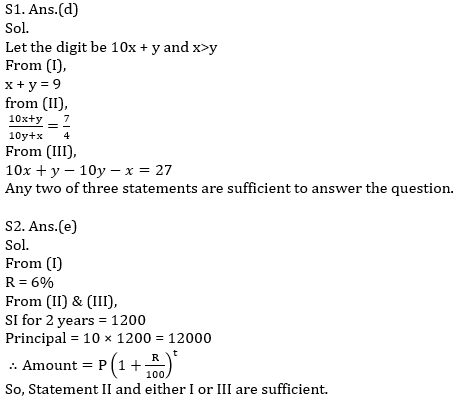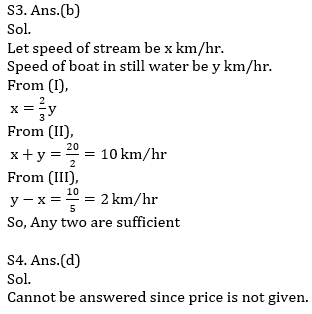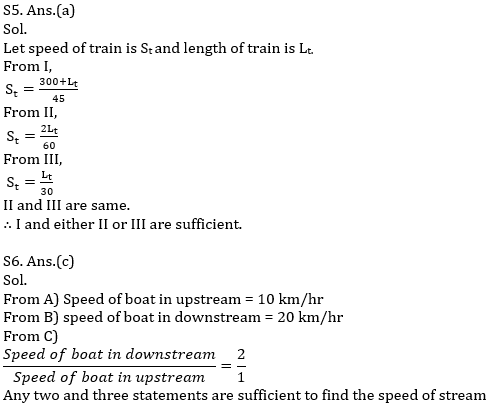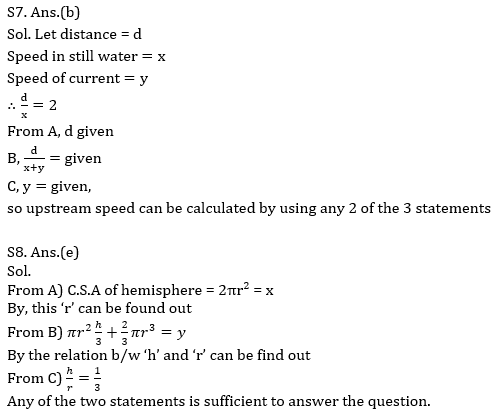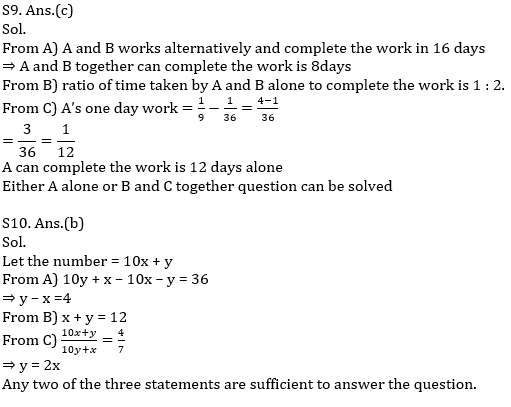Click Here to Register for Bank Exams 2021 Preparation Material#### Congratulations!Download Hindu Review of October 2021: Free PDF